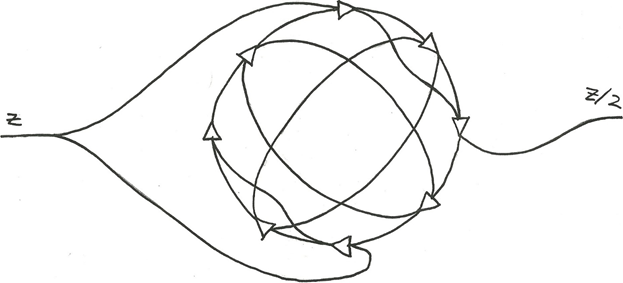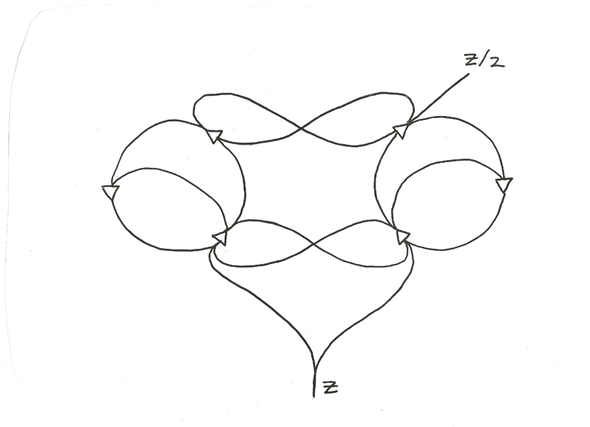## Wednesday, May 23, 2018

### Diamond Bracket Forms and How to Count to Two; 8 of 10

From "Cybernetics & Human Knowing", Vol. 24 (2017), No. 3-4, pp. 161-188

# 7. How to Count to Two

Here is the “First Brownian Modulator”:

_________________________________________
___________________________________     |
_____________________________     |     |
_______________________     |     |     |
_________________     |     |     |     |
_____________   |     |     |     |     |
_________   |   |     |     |     |     |
_____   |   |   |     |     |     |     |
| | | | | | |     |  |  |   | |  |  |
| Z |_|_|_| | | | Z   |__|__|___| |  |  |
|     | |_____|_|________|  |        |  |
|     |       | |___________|________|  |
|     |_______|_____________|           |
|_____________|_________________________|

It is equivalent to the bracket-form system:

Z  =   input
B  =   [ KZ ]
C  =   [ BD ]
D  =   [ BE ]
E  =   [ DF ]
F  =   [ HZ ]
G  =   [ FE ]
H  =   [ KC ]
K  =   [ HG ]G. Spencer-Brown, in his Laws of Form, claims that this circuit “counts to two”; i.e. when Z oscillates twice between marked and unmarked, K oscillates once.  Soon we will diffract this circuit, to reveal a circular “rotor” circuit.

Here is a “Naive Modulator”:

a   =    [ hz ]
b   =    [ gz ]
c   =    [ ad ]
d   =    [ bc ]
e   =    [ c [z] ]
f    =   [ d [z] ]
g   =    [ eh ]
h   =    [ fg ]

And here is the “Kauffman Modulator”:
a  =  [bjz]
b  =  [aiz]
c  =  [ad]
d  =  [bc]    =    z/2 ;   the half-period oscillator
i  =  [bd]
j  =  [ac]